Next: Solution of Inhomogeneous Wave Up: Maxwell's Equations Previous: Dirac Delta Function

# Three-Dimensional Dirac Delta Function

The three-dimensional Dirac delta function,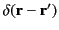, has the property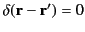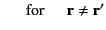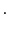(21)

In addition, however, the function is singular at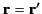in such a manner that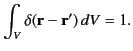(22)

Here,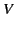is any volume that contains the point. (Also,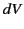is an element ofexpressed in terms of the components of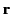, but independent of the components of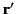.) It follows that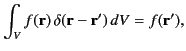(23)

where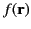is an arbitrary function that is well behaved at. It is also easy to see that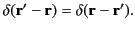(24)

We can show that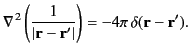(25)

(Here,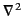is a Laplacian operator expressed in terms of the components of, but independent of the components of.) We must first prove that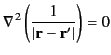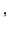(26)

in accordance with Equation (21). If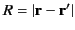then this is equivalent to showing that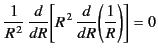(27)

for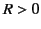, which is indeed the case. (Here,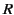is treated as a radial spherical coordinate.) Next, we must show that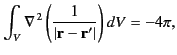(28)

in accordance with Equations (22) and (25). Suppose that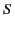is a spherical surface, of radius, centered on. Making use of the definition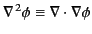, as well as the divergence theorem, we can write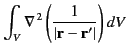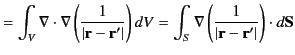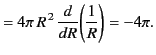(29)

(Here,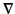is a gradient operator expressed in terms of the components of, but independent of the components of. Likewise,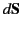is a surface element involving the components of, but independent of the components of.) Finally, ifis deformed into a general surface (without crossing the point) then the value of the volume integral is unchanged, as a consequence of Equation (26). Hence, we have demonstrated the validity of Equation (25).Next: Solution of Inhomogeneous Wave Up: Maxwell's Equations Previous: Dirac Delta Function
Richard Fitzpatrick 2014-06-27